﻿ Wreck Tangled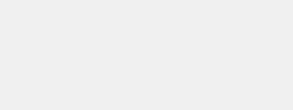Click to read general information about afghansBuy nowOther places to visit

Order Form

Mental Blocks

The World of Illusion Knittingfor this afghan

on the order form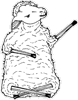Buy now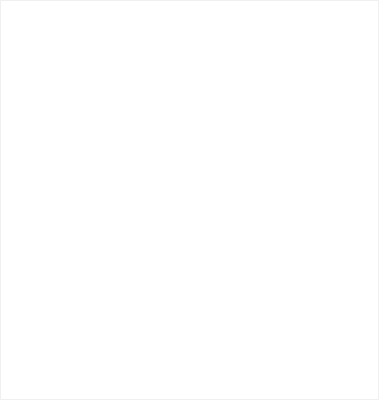WRECK TANGLED

The design consists of 14 different rectangles, all with the same area. The total area of the rectangles is equal to the total area of the background.

It may be difficult to believe that the long thin rectangles have the same area as the almost square ones.

Wreck Tangled always attracts interest at exhibitions specifically for mathematicians but seems to lack excitement for knitters. Perhaps the colours should be more striking to make it a ‘work of art’ as well as a mathematical curiosity.

The colours were deliberately chosen to be very much alike because the rectangles are much more alike than they may appear at first glance. Each rectangle has exactly the same area. There are the same number of stitches in every rectangle. The table below is based on the number of stitches in the width and the number of ridges. The actual number of stitches is actually twice this because two rows make one ridge.

In addition, the overall size of the afghan is 240 x 196 = 47040, which is exactly double the area of the rectangles. The background is, therefore, equal in area to all of the rectangles.Wreck Tangled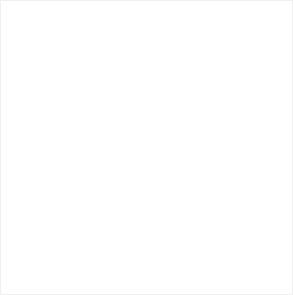RELATED DESIGNS

HALF-AND-HALF AFGHANS

Tilting at Windmills

Half Measures

HALF-AND-HALF CUSHIONS

Tilting at Windmills

Square is it?

Wreck TangledCONSTRUCTION INFORMATION

Knitted in one piece, using one yarn at a time. Various sections need to be left on stitch-holders for picking up at a later stage.

There is a very small amount of stitching to be done.KNITTING INFORMATION

The rectangles can all be made in the same colour or in up to fourteen different colours. The design is good for using up oddments.

In knitting terms, the equal areas of the rectangles mean that each rectangle has the same number of stitches and uses exactly the same amount of yarn.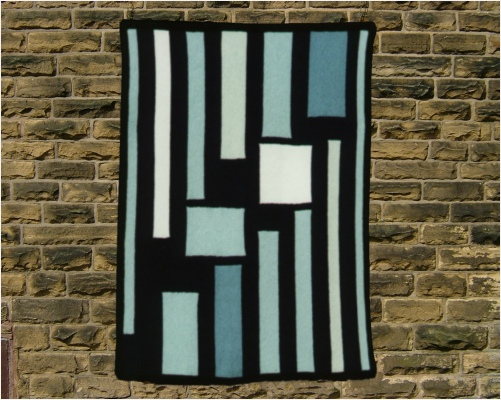240 x 7 = 1680 210 x 8 = 1680 168 x 10 = 1680 140 x 12 = 1680 120 x 14 = 1680 112 x 15 = 1680 105 x 16 = 1680 84 x 20 = 1680 80 x 21 = 1680 70 x 24 = 1680 60 x 28 = 1680 56 x 30 = 1680 35 x 48 = 1680 40 x 42 = 1680